December 2, 2021

# Class 10 Maths Chapter 7 Coordinate Geometry – Formulas & Notes PDF

We were introduced to the concept of the graph in class 9th, where we learned the very basics of the graph, and how to mark coordinates on a graph. In class 10 maths chapter 7 Coordinate Geometry, we will learn how one can find the distance between two points when the coordinates of the distance are given. Also, we will discover ways to find the area of a triangle that is formed by three points. All this will be thought with the help of different formulas like distance formula, section formula, area of a triangle, etc.

Since this is a graph-related chapter, therefore, general knowledge of graph is necessary to understand this chapter. This is why we will first go through all the important points that we had learned in class 9th and then move on to the advanced concept of class Xth.

## Class 10 Maths Chapter 7 Coordinate Geometry

Here is a quick summary of coordinate geometry from the previous class based on which we will build our understanding of coordinate geometry for class 10th.

• If we want to locate the position of a point in a plane, then we need a horizontal and a vertical line perpendicular to each other.
• The plane on which the lines are drawn is called the cartesian or coordinate plane and the lines are called the coordinate axes. Note that ‘axes’ is plural for ‘axis’.
• The vertical line on the cartesian plane is called the y-axis, and the horizontal line is known as the x-axis.
• Both these lines have positive and negative values. The section above origin has positive values, whereas the section below the origin has negative values. The section on the left of origin has negative values, and the section on the right of the origin has positive values.
• Both these axes divide the coordinate plane into four different sections called quadrants.
• The point on the cartesian plane at which both these axes intersect each other is called the origin.
• The distance of a point from the x-axis is called y-coordinate which is also known as ordinate.
• The distance of a point from the y-axis is called the x-coordinate which is also known as abscissa.
• Let the abscissa and ordinate of a point be x and y respectively, then (x, y) will be called the coordinates of that point. Note that while writing coordinates, the abscissa is written first and then ordinate.
• If the point lies on the x-axis then its coordinates will be (x, 0), since in this situation y is 0.
• If the point is on the y-axis, then its coordinates will be (0, y), since x is 0.
• The coordinates of the origin are always (0,0).
• If a point lies on the first quadrant on the cartesian plane, then its coordinates will be in the form (+,+), on the second quadrant it will be (-, +), on the third quadrant (-, -), and on the fourth (+, -). Here + denotes a positive real number and – denotes a negative real number.
• If x≠y, then (x, y) ≠ (y, x), and (x, y) = (y, x), if x = y.

## Class 10 Maths Chapter 7 Coordinate Geometry – Explanation Part 1

We now have all the important knowledge that is needed to understand class 10 maths chapter 7 coordinate geometry, therefore, we can now proceed to study this chapter in detail. So, let’s jump to an in-depth analysis of chapter 7.

# Distance formula

In coordinate geometry, the distance formula is used to get the distance between two points, for which the coordinates are known to us. So, if we are to find the distance between two points (P & Q) whose coordinates are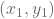and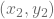is given by: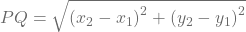.

# Section Formula

For class 10 maths chapter 7 coordinate geometry, consider that we are to find the coordinate of a point that divides a line segment that joins two points into two parts such that the ratio of their length is m:n. This can be done with the section formula.

Let there be a line segment joining the points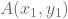and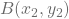. Now there is a point P(x, y) that internally divides this line segment in the ratio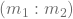, then the section formula for this will be given as: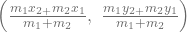If the ratio in which P divides AB is k:1, then the coordinates of the point P will be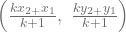Finding coordinates of the mid-point: if a point divides a line segment in 1:1 ratio, then formula to find the coordinates of such point is given by: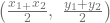Class 10 Maths Chapter 7 Coordinate Geometry – Explanation Part 2

For the final part of this chapter, we will discuss how to find the area of a triangle using coordinate geometry.

# Area of triangle

We know that area of a triangle is given by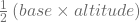, therefore, for a given triangle whose vertices and their coordinates are,, and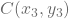, then the formula to calculate it’s the area will be given as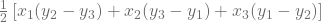So these are all the important formulas for class 10 maths chapter 7 coordinate geometry. Now let’s have a look at the blueprint of chapter 7.

Class 10 maths chapter 7 coordinate geometry blueprint
1 Marker 1 question
2 Marker 1 question
4 Marker 1 question
Total questions 3
Total marks 7

In the board exam, there will be a total of 3 questions from this chapter worth 7 marks. Out of the 3 questions, 1 question will be one marker, while 1 question will be 2 markers, and 1 question will be of 4 markers.

Class 10 maths chapter 7 coordinate geometry is comparatively a bit easy to cover making it a scoring chapter. So, make sure to prepare it well to get the maximum out of this chapter.